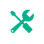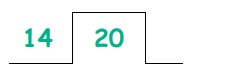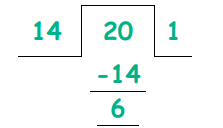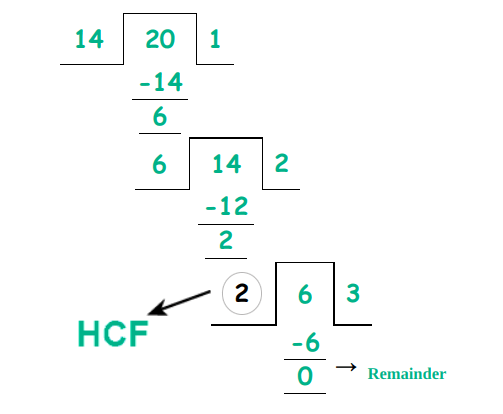# GCF CALCULATOR

How it Works?
1. 1. Enter numbers in first input box separated by comma
2. 2. Select Calculation Method from dropdown
3. 3. Click on calculate button
GCF of
302
Finding GCF by Using List of factors Method
Finding GCF by Using Division Step Method
Finding GCF by Using Prime factorization Method

## LCM Calculator

The online LCM calculator can find the least common multiple (factors) quickly than manual methods. Whether finding the LCM of two numbers or multiple numbers, this calculator can help you with just a single click.Related Tool Name

Greatest Common Factor aka Greatest Common Divisor calculator is an online maths tool that finds the GCF of two or several numbers by all three GCF methods.

Through GCF (GCD) calculator, you can select the method of your choice. The GCF calculation through the selected method will be shown with a detailed explanation.

You can simply click on the other method to see its process, that is, you will not have to enter the values all over from the start.

## What is GCF?

Short form for Greatest Common Factor, GCF is the one factor that has the highest value among the common factors.

Other names of GCF include HCF and GCD.

## How to find GCF?

It is a little tricky to find GCF, that is why the GCF finder above is recommended. But for your information and concept, we will see how to find GCF in detail.

The famous methods used for calculating GCF are:

• Prime factorization
• Division method
• List of factors

You can see the example of prime factorization in the HCF and LCM calculator. In this post, we will learn the remaining two methods through examples.

### 1. Division method:

Example:

Find the GCF of 14 and 20 through the division method.

Solution:

Step 1: Use the higher value as a dividend and the lower value as the divisor.

Larger value = 20

Lower value = 14Step 2: Divide by the nearest multiple.

The nearest value to 20 in 14’s time's table is 14 itself.Step 3: Continue until no more can be solved. The last divisor is GCF (HCF).### 2. List of Factors:

Example:

What is the greatest common factor of 12, 16, 29? Use the list of factors method.

Solution:

Step 1: Write all the factors of 12, 16, and 29

Factors of 12 = 1, 2, 3, 4, 6, 12.

Factors of 16 = 1, 2, 4, 8, 16.

Factors of 29 = 1, 29.

Step 2: Look for the common factors.

There is only one common factor among these three numbers i.e 1

Step 3: Identify the greatest common factor.

Since there is no other factor, 1 will be the GCF of 12, 16, and 29.